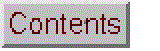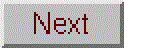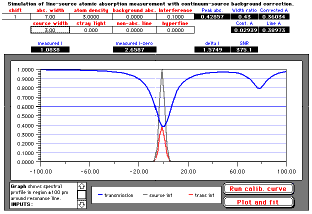# Spectroscopic Simulation of Atomic Absorption

 Excel version Calc versionOld WingZ version

Click to see larger view

This is a simulation of the spectroscopy of a line-source atomic absorption (AA) measurement.  It is not a simulation of an AA instrument.  What's the difference?  An instrument simulation shows you how to work an AA instrument.  This simulation shows you how AA spectroscopy works, that is, what goes on under the instrument's surface. So much is hidden from the observer in AA spectroscopy; with a typical line-source instrument, it is not even possible to scan the spectrum of the light source or the absorber with sufficient resolution to see what is going on.

The purpose of the simulation is to make it clearer how the various spectroscopic aspects relate to each other and to the measured absorbance, in a line-source atomic absorption measurement with continuum-source background correction in a steady-state (i.e. flame) atomizer. You can observe the spectral  relationship of the hollow cathode lamp emission profile to the atomic absorption profile, observe the effect of different spectral line widths of both the absorbing atom and the hollow cathode lamp, correction of background absorption by continuum-source (D2) method, over-correction caused by structured background absorption, and the effect of non-absorbing lines, line-overlap interferences, and hyperfine structure.

Several simplifying assumptions are made, which do not impact the main points to be made. In this simulation, the atomic absorption lines are modeled as a Lorentzian (although the actual line shape depends on the relative contributions of Doppler and collisional (pressure) broadening, both of which temperature and pressure dependent. The hollow cathode lamp's line is modeled as a Gaussian (temperature-broadened only, not pressure broadened, due to the low internal pressure of the lamp). Background absorption is assumed to be constant over the spectral bandwidth of the spectrometer, which is fixed at 0.2 nm. The simulation is meant to be generic for any typical element, not tailored to the specific spectroscopic properties of any one element. Hence, the wavelength scale (x-axis) is relative to the atomic line center and extends positive and negative from that.

AtomicAbsorption.xlsx for Excel
AtomicAbsorption2.ods for OpenOffice Calc
AtomicAbsorption.wkz; for WingZ
(Wingz player application and basic set of simulation modules, for windows PCs or Macintosh)

### Cell Equations and Definitions (WingZ version):

`INPUTS:shift = collisional shift of absorption line, pm.abs. width = spectral width of atomic absorption line, pm.source width = spectral width of hollow-cathode lamp     emission line, pm.atom density = relative concentration of atoms in atomizer,     arbitrary units.stray light = relative intensity of continuum background radiation     from hollow-cathode lamp.background abs. = non-specific background absorption in atomizer.non-abs. line = intensity of a non-absorbing line from HCL.    arbitrarily placed at -60 pm, relative to main resonance line.interference = peak absorbance of matrix absorption line,        arbitrarily placed at +60 pm.hyperfine = relative intensity of hyperfine line,     relative to main resonance line.Array calculations:A39..A139: wavelength = -100 to +100 (displacement in pm from    resonance wavelength)Total number of wavelength intervals = NumWavelengthsB39..B139: absorbance = PeakAbs/(1+((wavelength-shift)/AbsWidth)^2)+ Interf/(1+((wavelength-80)/AbsWidth)^2)+ Hyper*PeakAbs/(1+((wavelength-6)/AbsWidth)^2) C39..C139: transmission = 10^(-absorbance-BackAbs) D39..D139: SourceIntensity   = exp(-((wavelength) / SourceWidth)^2) + StrayLight/100+ NonAbs*exp(-((wavelength+60)/SourceWidth)^2)+ Hyper*exp(-((wavelength-6)/SourceWidth)^2) E39..E139: TransmittedIntensity = transmission*SourceIntensityGraph shows spectral profile in region ±100 pm around resonance line. Gray line: SourceIntensity Blue line: transmission Red line: TransmittedIntensity OUTPUTS:Peak abs. = "true" peak absorbance at center of absorption line.Width ratio = ratio of absorption width to source width.Cont. A = absorbance measured with continuum source.Uncorr. A = absorbance measured with line source.Corrected A = atomic absorbance corrected for background absorbance   (equals Uncorr. A - Cont. A).measured I = total intensity transmitted through atomizer, measured    at the detector over the entire spectral bandpass.measured I-zero = total incident intensity measured at detector    over entire spectral bandpass.delta I = difference between measured I-zero and measired I.SNR = signal-to-noise ratio for photon-limited measurement.Display calculations:measured I = MeasI = sum(TransmittedIntensity) measured I-zero = MeasIzero = sum(SourceIntensity) delta I = MeasIzero-MeasI SNR = 1000*CorrectedA*sqrt(MeasI)Peak abs. = Conc/(AbsWidth) Width ratio = SourceWidth/AbsWidth Cont. A = Ac =log((NumWavelengths))/(sum(transmission))) Line A = Al = log(MeasIzero/MeasI)CorrectedA = Al-AcOperating Instructions`

### Computer Simulation of the Spectroscopy of Atomic Absorption

The graph displays a plot of intensity on the y axis vs wavelength displacement from the resonance wavelength, in pm, on the x axis. The x axis extends over the spectral bandpass of the monochromator (0.2 nm, or 200 pm, in this case), which is centered on the resonance wavelength. There are three lines on the plot in different colors: the spectral profile of the incident line-source emission line (gray), the analyte transmission profile (blue), and the spectral profile of the transmitted line-source emission line (red). (In a real AA instrument you actually wouldn't be able to see these spectral profiles, so in that respect this simulation is more instructive that a real instrument). The purpose of the simulation is to make it clearer how the various spectroscopic aspects relate to each other and to the measured absorbance. In particular, this simulation assumes an instrument with a continuum- source background correction in a steady-state (i.e. flame) atomizer. The input parameters that you have direct control over are displayed at the top of the screen in the boxed cells with red labels:

 shift Collisional ("red") shift of the absorption line, pm. abs. width Spectral width of the the analyte's atomic absorption line, pm. source width Spectral width of the hollow-cathode lamp emission line, pm. atom density Relative atom density of analyte atoms, arbitrary units. stray light Relative intensity of continuum background radiation from the hollow-cathode lamp. background abs. Absorbance of the non-specific background absorption in the atomizer non-abs. line Intensity of a non-absorbing line from the HCL, arbitrarily placed at -60 pm, relative to the main resonance line. interference Peak absorbance of a matrix absorption line, arbitrarily placed at +60 pm, relative to the main resonance line. hyperfine Relative intensity of the hyperfine line, relative to intensity of the "main" line.

To change any of these parameters, click on the number, type in a new value, and press the ENTER key. Recalculation is automatic.

The calculated "outputs" are shown in the black boxes with white numbers and blue labels:

 Peak abs. The "true" peak absorbance at the center of the absorption line. Width ratio Ratio of the absorption width to the source width. Cont. A Absorbance measured with the continuum source. Line A Absorbance measured with the line source. Corrected A Atomic absorbance corrected for background absorbance. (This is simply equal to Line A - Cont. A). measured I Total intensity transmitted through the atomizer measured over the entire spectral bandpass. measured I-zero Total incident intensity measured over the entire spectral bandpass. delta I Difference between measured I-zero and measured I. SNR Theoretical signal-to-noise ratio for photon-noise-limited measurement.

Of course in a real AA instrument you wouldn't get all these outputs: usually only the uncorrected and corrected absorbances are displayed. On some instruments you can read the background absorbance (Cont A) and the intensities (measured I or measured I-zero). No instrument displays the true peak absorbance (it's fundamentally unknown), the width ratio, or the theoretical SNR.

There are also two buttons that automatically acquire an analytical curve:

 Run calib. curve Varies the atom density from 0 to 10 units in steps of 1 and records the corrected absorbance. Plot and fit Plots the resulting analytical calibration curve on a separate sheet, fits a straight line to the low absorbance (linear) region, and displays the slope and intercept of the line.

If the analytical curve is linear over the range of expected sample concentrations, then you can calibrate the instrument by running a single standard solution to determine the proportionality constant between concentration and absorbance, then use that factor to convert the absorbance readings on unknown samples into concentration.  If the analytical curve is not linear over the range of expected sample concentrations, then you must calibrate the instrument by running a series of standard solutions over that range range and plotting absorbance vs standard concentration.  This curve is then used to convert the absorbance readings on unknown samples into concentration.

Note than this simulation does not have a slit width control. Line-source atomic absorption is different than other forms of absorption spectroscopy because the primary light source is an atomic line source (e.g. hollow cathode lamp or electrodeless discharge lamp) whose spectral width is not a function of the monochromator slit width, but rather is controlled by the temperature and pressure within the lamp and by the hyperfine splitting of that element.  The only control that the operator has over the source line width is the operating current of the lamp; increased current causes increased temperature and pressure, both of which lead to increased line width (increased source width), as well a higher lamp intensity.  So, to that extent, the effect of increasing the lamp current is a bit like the effect of increasing the slit width in a continuum-source absorption instrument; the intensity goes up (good) but the increased source width and increasing polychromatic effect causes calibration non-linearity (bad).  The slit width on a line-source atomic absorption instrument is also adjustable by the user, but it has no effect on the source width, being much greater (by 1000-fold or so) than the actual line width of the atomic line source.  (Typical atomic line width 0.001 - 0.003 nm, compared to typical spectral bandpass of 1 nm). Increasing the slit width does increase the light intensity linearity (because the entrance slit area increases directly), but operating a too large a slit width runs the risk of increasing the stray light, by allowing in other lines emitted by the atomic line source that are not absorbed by the analyte in the atomizer, such as lines originating from the fill gas, impurities in the lamp, and non-resonance lines of the analyte.  As always, stray light leads to non-linearity, especially at high concentrations and eventually to a plateau in the calibration curve at high absorbances.

Student Assignment

Open the file "AAMeasurement.wkz".

1. Make sure that all the input parameters are set to their default values: shift =1, abs. width = 3.5, source width = 1, and atom density = 1, and all other inputs = 0. (These source and absorption widths are typical values for the Ca 422 nm resonance line).

2. Note that the line source absorbance (Line A) is slightly lower that the Peak abs. Why? Does this lead to inaccuracy in analytical applications?

3. Increase atom density to 10. Note that Peak abs. increases by exactly 10 as it should. Did the line source absorbance (Line A) also increase by ten-fold? Why not?

4. Note that the Corrected A is lower that Line A, because the continuum source absorbance Cont. A is not zero. Why is this so? To check that it is not an offset or zero-adjust problem, set atom density to zero and verify that all absorbances are zero. Does this have an effect on the analytical linearity also? To test that, compare the corrected and uncorrected absorbances at atom density of 1 and 10.

5. Return the atom density to 1. To demonstrate that background correction is necessary, increase the background abs. and notice the effect on Cont. A, Corrected A and Line A. (In this idealized simulated instrument, misalignment between the hollow cathode and continuum lamps is not included, so the correction is perfect; it is never this perfect in practice). Note that although the correction is perfect, the SNR degrades (decreases) when the background absorbance increases. Why?

6. Return the background abs. to 0 and increase the interference, which controls the peak absorbance of a matrix absorption line that falls within the spectral bandpass but does not overlap the analytical absorption line. Note that the Line A is not much changed, but the Cont. A, and thus the Corrected A, is. Why? If only this kind of interference occurs in a particular application, would it be more accurate to use the corrected or uncorrected (line) absorbance for analytical purposes? How do the Zeeman and pulsed HCL methods minimize this problem?

7. Return the interference to 0. Set non-abs line to 0.1. This controls the relative intensity of a nonresonance (non-absorbing) line emitted by the hollow cathode lamp that falls within the spectral bandpass. Note the effect on the absorbances. Click on the Run calib. curve button and, when it is finished running through all the atom densities, click on the Plot and fit button to see the resulting analytical curve. Explain the shape of the analytical curve. (Note: each analytical curve plot is placed in a separate window, to allow two or more to be compared; to discard them, click in the close box in the upper left, the click in NO on the following dialog box).

8. Return the non-abs line to 0. Set hyperfine to 0.5 and notice the effect of the transmission profile and the source line profile. Do an experiment to determine if the presence of hyperfine structure influences the sensitivity or linearity. What happens if hyperfine = 1? 9. Return hyperfine to 0. All parameters should now be back at their default values. Determine by experiment the absorbance that gives the best SNR. Do your results agree with Ingle and Crouch for a photon (shot) noise limit? See page 284 of James D. Ingle, Jr. and Stanley R. Crouch, Spectrochemical Analysis, Prentice Hall, New Jersey, 1988.

(c) 1991, 2015. This page is part of Interactive Computer Models for Analytical Chemistry Instruction, created and maintained by Prof. Tom O'Haver, Professor Emeritus, The University of Maryland at College Park.
Comments, suggestions and questions should be directed to Prof. O'Haver at toh@umd.edu.